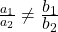# TS Polycet (Polytechnic) 2018 Previous Question Paper with Answers And Model Papers With Complete AnalysisTS Polycet (Polytechnic) 2018 Previous Question Paper with Answers And Model Papers With Complete Analysis
TS Polycet (Polytechnic) Previous Year Question Papers And Model Papers:
While preparing for TS Polycet (Polytechnic), candidates must also refer to the previous year question papers of the same. Scoring well in TS Polycet (Polytechnic) and understanding weaknesses and strengths in the respective sections.

TS Polycet (Polytechnic) Previous Year Question Papers can be found on this page in PDF format. Students taking the exam to get into some of the best Polytechnic colleges/institutes in the state of Andhra Pradesh may practice these papers to get a clear idea of the structure of the exam, marking scheme, important topics, etc.

## Section — I MATHEMATICS

Q). ab (c) = a (bc) is called property.
A) Associative
B) Inverse
C) Identity
D) None

A) Associative

Q). √5 +√7 is ……………….. number.
A) Natural
B) Rational
C) Integer
D) an Irrational

D) an Irrational
Explanation: Sum of two irrational numbers is an irrational number.

Q). (log9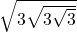) log10 0.01 =
A) (7/8)1
B) (7/16)0
C) (1/16)-2
D) (1/8)2

Explanation: A, B, C, D

Q). Exponential form of log464 = 3 is
A) 34 = 64
B) 43 = 64
C) 42 = 81
D) None

C) 42 = 81
Explanation: log464 = 3 ⇒ 43 = 64

Q).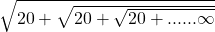=
A) 1
B) 3
C) 5
D) 6

C) 5
Explanation: Let x =X =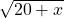⇒ x2 = 20+x
x2 -x – 20 = 0 => x2 – 5x + 4x – 20 = 0
x(x-5) + 4(x-5) = 0=> (x-5) (x + 4) = 0
=> x = 5

Q). From the following Venn-diagram B – A =…………………….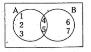A) {1,2, 3}
B) {4, 5}
C) {6, 7}
D) None

C) {6, 7}
Explanation: From the Venn-diagram
B -A = {4,5,6,7} -{1, 2,3,4,5}
= {6, 7}

Q). If A = {a, b, c, d}, then the number of subsets for A is …………………….
A) 5
B) 6
C) 16
D) 65

C) 16
Explanation: Given A = {a, b, c, d} => n(A) = 4

No. of subsets = 2n = 24 = 16

Q). If α,β,ɣ are the zeroes of the polynomial
f(x) = ax3 + bx2 + cx + d, then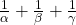A) 1/d
B) 1/c
C) c/d
D) –c/d

D) –c/d
Explanation: Given f(x) = ax3 + bx2 + cx + d
==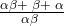=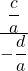=-c/d

Q). A quadratic polynomial whose zeroes are 5 and -2 is ………………
A) x2 + 5x-2
B) x2 + 3x-10
C) x2-3x-10
D) x2-2x + 5

C) x2-3x-10
Explanation: α = 5 β = -2
x2 – (α + β) X + αβ = 0
⇒ x2 – (5 – 2) x + 5 (-2) = 0
⇒ x2-3x-10 = 0

Q). The lines represented by 5x + 7y – 14 = 0 and 10x + 3y – 8 = 0 are…….. lines.
A) Coincident
B) Vertical
C) Parallel
D) Intersecting